Home | | Physics 11th std | BernoulliŌĆÖs Theorem

# BernoulliŌĆÖs Theorem

In order to discuss the mass flow rate through a pipe, it is necessary to assume that the flow of fluid is steady, the flow of the fluid is said to be steady if at any given point, the velocity of each passing fluid particle remains constant with respect to time.

BERNOULLIŌĆÖS THEOREM

## Equation of continuity

In order to discuss the mass flow rate through a pipe, it is necessary to assume that the flow of fluid is steady, the flow of the fluid is said to be steady if at any given point, the velocity of each passing fluid particle remains constant with respect to time. Under this condition, the path taken by the fluid particle is a streamline.

Consider a pipe AB of varying cross sectional area a1 and a2 such that a1 > a2. A non-viscous and incompressible liquid flows steadily through the pipe, with velocities v1 and v2 in area a1 and a2, respectively as shown in Figure 7.32.Let m1 be the mass of fluid flowing through section A in time ╬öt, m1 = (a1v1 t) Žü

Let m2 be the mass of fluid flowing through section B in time t, m2 = (a2v2 t) Žü

For an incompressible liquid, mass is conserved m1 = m2

a1 v1 ╬öt Žü = a2 v2 ╬öt Žüwhich is called the equation of continuity and it is a statement of conservation of mass in the flow of fluids.

In general, a v = constant, which means that the volume flux or flow rate remains constant throughout the pipe. In other words, the smaller the cross section, greater will be the velocity of the fluid.

## EXAMPLE 7.14

In a normal adult, the average speed of the blood through the aorta (radius r = 0.8 cm) is 0.33 ms-1. From the aorta, the blood goes into major arteries, which are 30 in number, each of radius 0.4 cm. Calculate the speed of the blood through the arteries.

### Solution:

a1v1 = 30 a2 v2 ŌćÆ ŽĆ r12v1  = 30 ŽĆ r22v2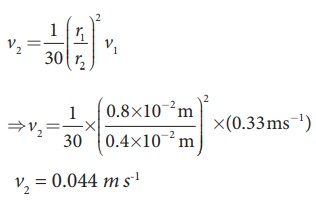## Pressure, kinetic and potential energy of liquids

A liquid in a steady flow can possess three kinds of energy. They are (1) Kinetic energy, (2) Potential energy, and (3) Pressure energy, respectively.

i) Kinetic energy:ŌĆé The kinetic energy of a liquid of mass m moving with a velocity v is given byThe kinetic energy per unit mass =Similarly, the kinetic energy per unit volumeii) Potential energy: The potential energy of a liquid of mass m at a height h above the ground level is given by

PE = mgh

The potential energy per unit massSimilarly, the potential energy per unit volume =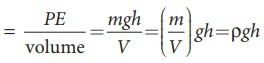ii) Pressure energy: The energy acquired by a fluid by applying pressure on the fluid. We know that

Pressure = Force/Area ŌćÆ Force = Pressure ├Ś Area

F ├Ś d = (P A) ├Ś d = P (A ├Ś d)

F ├Ś d = W = P V = pressure energy

Therefore, pressure energy, EP = PV The pressure energy per unit mass =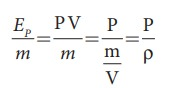Similarly,  the  potential  energy  per  unit volume =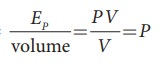## BernoulliŌĆÖs theorem and its applications

In 1738, the Swiss scientist Daniel Bernoulli developed a relationship for the flow of fluid through a pipe of varying cross section. He proposed a theorem for the streamline flow of a liquid based on the law of conservation of energy.

## BernoulliŌĆÖs theorem

According to BernoulliŌĆÖs theorem, the sum of pressure energy, kinetic energy, and potential energy per unit mass of an incompressible, non-viscous fluid in a streamlined flow remains a constant. Mathematically,This is known as BernoulliŌĆÖs equation.

### Proof: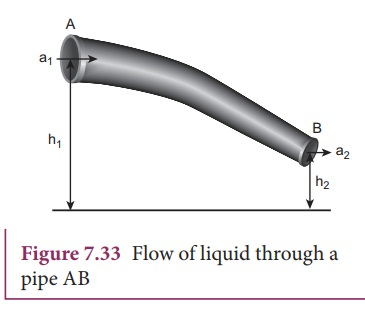Let us consider a flow of liquid through a pipe AB as shown in Figure 7.33. Let V be the volume of the liquid when it enters A in a time t which is equal to the volume of the liquid leaving B in the same time. Let aA, vA and PA be the area of cross section of the tube, velocity of the liquid and pressure exerted by the liquid at A respectively.

Let the force exerted by the liquid at A is

FA = PAaA

Distance travelled by the liquid in time t is

d = vA t

Therefore, the work done is

W = FAd = PAaAvA t

But aAvAt = aAd =V, volume of the liquid entering at A.

Thus, the work done is the pressure energy (at A),ŌĆāSince m is the mass of the liquid entering at A in a given time, therefore, pressure energy of the liquid at A is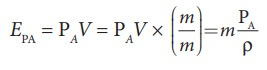Potential energy of the liquid at A,

PEA = mg hA,

Due to the flow of liquid, the kinetic energy of the liquid at A,Therefore, the total energy due to the flow of liquid at A, EA = EPA + KEA + PEASimilarly, let aB, vB, and PB be the area of cross section of the tube, velocity of the liquid, and pressure exerted by the liquid at B. Calculating the total energy at EB, we getFrom the law of conservation of energy,

EA = EBThus, the above equation can be written asThe above equation is the consequence of the conservation of energy which is true until there is no loss of energy due to friction. But in practice, some energy is lost due to friction. This arises due to the fact that in a fluid flow, the layers flowing with different velocities exert frictional forces on each other. This loss of energy is generally converted into heat energy. Therefore, BernoulliŌĆÖs relation is strictly valid for fluids with zero viscosity or non-viscous liquids. Notice that when the liquid flows through a horizontal pipe, then h = 0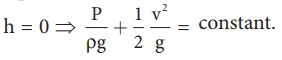## Applications of BernoulliŌĆÖs Theorem

(a) Blowing off roofs during wind storm In olden days, the roofs of the huts or houses were designed with a slope as shown in Figure.7.34. One important scientific reason is that as per the BernoulliŌĆÖs principle, it will be safeguarded except roof during storm or cyclone.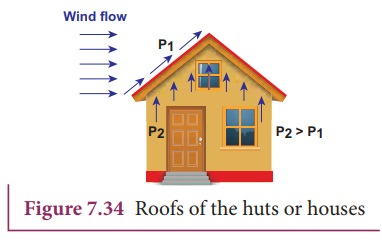During cyclonic condition, the roof is blown off without damaging the other parts of the house. In accordance with the BernoulliŌĆÖs principle, the high wind blowing over the roof creates a low-pressure P1. The pressure under the roof P2 is greater. Therefore, this pressure difference (P2ŌĆōP1) creates an up thrust and the roof is blown off.

### (b) Aerofoil lift

The wings of an airplane (aerofoil) are so designed that its upper surface is more curved than the lower surface and the front edge is broader than the real edge. As the aircraft moves, the air moves faster above the aerofoil than at the bottom as shown in Figure 7.35.

According to BernoulliŌĆÖs Principle, the pressure of air below is greater than above, which creates an upthrust called the dynamic lift to the aircraft.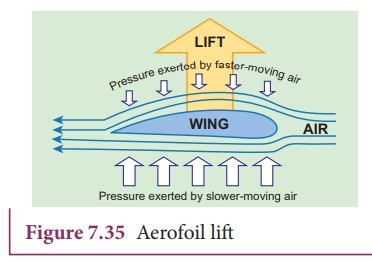### (c) Bunsen burner

In this, the gas comes out of the nozzle with high velocity, hence the pressure in the stem decreases. So outside air reaches into the burner through an air vent and the mixture of air and gas gives a blue flame as shown in Figure 7.36.### (d) Venturimeter

This device is used to measure the rate of flow (or say flow speed) of the incompressible fluid flowing through a pipe. It works on the principle of BernoulliŌĆÖs theorem. It consists of two wider tubes A and A' (with cross sectional area A) connected by a narrow tube B (with cross sectional area a). A manometer in the form of U-tube is also attached between the wide and narrow tubes as shown in Figure7.37. The manometer contains a liquid of density ŌĆśŽümŌĆÖ.Let P1 be the pressure of the fluid at the wider region of the tube A. Let us assume that the fluid of density ŌĆśŽüŌĆÖ flows from the pipe with speed ŌĆśv1ŌĆÖ and into the narrow region, its speed increases to ŌĆśv2ŌĆÖ. According to the BernoulliŌĆÖs equation, this increase in speed is accompanied by a decrease in the fluid pressure P2 at the narrow region of the tube B. Therefore, the pressure difference between the tubes A and B is noted by measuring the height difference (╬öP = P1ŌłÆP2) between the surfaces of the manometer liquid.

From the equation of continuity, we can say that Av1 = a v2 which means that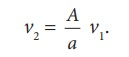Using BernoulliŌĆÖs equation,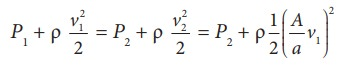From the above equation, the pressure differenceThus, the speed of flow of fluid at the wide end of the tube AThe volume of the liquid flowing out per second is### (e) Other applications

This BernoulliŌĆÖs concept is mainly used in the design of carburetor of automobiles, filter pumps, atomizers, and sprayers.

For example, the carburetor has a very fine channel called nozzle through which the air is allowed to flow in larger speed. In this case, the pressure is lowered at the narrow neck and in turn, the required fuel or petrol is sucked into the chamber so as to provide the correct mixture of air and fuel necessary for ignition process.

Tags : Equation, its applications , 11th Physics : UNIT 7 : Properties of Matter
Study Material, Lecturing Notes, Assignment, Reference, Wiki description explanation, brief detail
11th Physics : UNIT 7 : Properties of Matter : BernoulliŌĆÖs Theorem | Equation, its applications